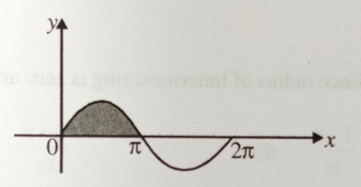A particle moves in the x-y plane with velocity ${\mathrm{v}}_{\mathrm{x}}=8\mathrm{t}-2$ and ${\mathrm{v}}_{\mathrm{y}}=2$. If it passes through the point x=14 and y=4 at t=2 sec. The equation of the path is

(A)  $\mathrm{x}={\mathrm{y}}^{2}-\mathrm{y}+2$

(B)  $\mathrm{x}=\mathrm{y}+2$

(C)  $\mathrm{x}={\mathrm{y}}^{2}+2$

(D)  $\mathrm{x}={\mathrm{y}}^{2}+\mathrm{y}+2$

Concept Questions :-

Integration
High Yielding Test Series + Question Bank - NEET 2020

Difficulty Level:

A motor boat of mass m moving along a lake with velocity ${\mathrm{V}}_{0}$. At t=0, the engine of the boat is shut down. Magnitude of resistance force offered to the boat is equal to rV. (V is instantaneous speed). What is the total distance covered till it stops completely?

(A)  ${\mathrm{mV}}_{0}/\mathrm{r}$

(B)

(C)  ${\mathrm{mV}}_{0}/2\mathrm{r}$

(D)  $2{\mathrm{mV}}_{0}/\mathrm{r}$

Concept Questions :-

Integration

Difficulty Level:

A particle is moving along positive x-axis. Its position varies as $\mathrm{x}={\mathrm{t}}^{3}-3{\mathrm{t}}^{2}+12\mathrm{t}+20$, where x is in meters and t is in seconds.

Initial velocity of the particle is

(A)  1 m/s

(B)  3 m/s

(C)  12 m/s

(D)  20 m/s

Concept Questions :-

Differentiation
High Yielding Test Series + Question Bank - NEET 2020

Difficulty Level:

A particle is moving along positive x-axis. Its position varies as $\mathrm{x}={\mathrm{t}}^{3}-3{\mathrm{t}}^{2}+12\mathrm{t}+20$, where x is in meters and t is in seconds.

Initial acceleration of the particle is

(A)  Zero

(B)

(C)

(D)

Concept Questions :-

Differentiation

Difficulty Level:

A particle is moving along positive x-axis. Its position varies as $\mathrm{x}={\mathrm{t}}^{3}-3{\mathrm{t}}^{2}+12\mathrm{t}+20$, where x is in meters and t is in seconds.

Velocity of the particle when its acceleration zero is

(A)  1 m/s

(B)  3 m/s

(C)  6 m/s

(D)  9 m/s

Concept Questions :-

Differentiation

Difficulty Level:

Two forces  and  are acting on a particle.

The resultant force acting on particle is:

(A)  $2\stackrel{^}{\mathrm{i}}+5\stackrel{^}{\mathrm{j}}+4\stackrel{^}{\mathrm{k}}$

(B)  $2\stackrel{^}{\mathrm{i}}-5\stackrel{^}{\mathrm{j}}-4\stackrel{^}{\mathrm{k}}$

(C)  $\stackrel{^}{\mathrm{i}}-3\stackrel{^}{\mathrm{j}}-2\stackrel{^}{\mathrm{k}}$

(D)  $\stackrel{^}{\mathrm{i}}-\stackrel{^}{\mathrm{j}}-\stackrel{^}{\mathrm{k}}$

Difficulty Level:

Two forces  and  are acting on a particle.

The angle between  is:

(A)  $\mathrm{\theta }={\mathrm{cos}}^{-1}\left(\frac{3}{2\sqrt{5}}\right)$

(B)  $\mathrm{\theta }={\mathrm{cos}}^{-1}\left(\frac{3}{5\sqrt{2}}\right)$

(C)  $\mathrm{\theta }={\mathrm{cos}}^{-1}\left(\frac{2}{3\sqrt{5}}\right)$

(D)  $\mathrm{\theta }={\mathrm{cos}}^{-1}\left(\frac{\sqrt{3}}{5}\right)$

Concept Questions :-

Resultant of Vectors

Difficulty Level:

Two forces  and  are acting on a particle.

The magnitude of the component of force ${\stackrel{\to }{\mathrm{F}}}_{1}$ along force ${\stackrel{\to }{\mathrm{F}}}_{2}$ is:

(A)  $\frac{5}{6}$

(B)  $\frac{5}{3}$

(C)  $\frac{6}{5}$

(D)  $\frac{5}{2}$

Concept Questions :-

Resultant of Vectors

Difficulty Level:

$\mathrm{A}=4\mathrm{i}+4\mathrm{j}-4\mathrm{k}$ and $\mathrm{B}=3\mathrm{i}+\mathrm{j}+4\mathrm{k}$, then angle between vectors A and B is:

(A)  $180°$

(B)  $90°$

(C)  $45°$

(D)  $0°$

Concept Questions :-

Resultant of Vectors

Difficulty Level:

A curve is governed by the equation y=sinx, then what is the area enclosed by the curve and x-axis between x =0 and x =$\mathrm{\pi }$ is (shaded region)1. 1 units

2. 2 units

3. 3 units

4. 4 units

Concept Questions :-

Integration